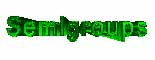Never thought that I would ever consider Galois descent of semigroup
coalgebras
but in preparing for my talks for the master-class it
came about naturally. Let A be a formally smooth algebra
(sometimes called a quasi-free algebra, I prefer the terminology
noncommutative curve) over an arbitrary base-field k. What, if
anything, can be said about the connected components of the affine
k-schemes rep(n,A) of n-dimensional representations
of A? If k is algebraically closed, then one can put a
commutative semigroup structure on the connected components induced by
the sum map

rep(n,A) x rep(m,A) -> rep(n + m,A)   :  (M,N)
-> M + N

as introduced and studied by Kent
Morrison
a long while ago. So what would be a natural substitute for
this if k is arbitrary? Well, define pi(n) to be the
maximal unramified sub k-algebra of k(rep(n,A)),
the coordinate ring of rep(n,A), then corresponding to the
sum-map above is a map

pi(n + m) -> pi(n) \\otimes
pi(m)

and these maps define on the graded
space

Pi(A) = pi(0) + pi(1) + pi(2) + ...

the
structure of a graded commutative k-coalgebra with
comultiplication

pi(n) -> sum(a + b=n) pi(a) \\otimes
pi(b)

The relevance of Pi(A) is that if we consider it
over the algebraic closure K of k we get the semigroup
coalgebra

K G  with  g -> sum(h.h\' = g) h \\otimes
h\'

where G is Morrison\’s connected component
semigroup. That is, Pi(A) is a k-form of this semigroup
coalgebra. Perhaps it is a good project for one of the students to work
this out in detail (and correct possible mistakes I made) and give some
concrete examples for formally smooth algebras A. If you know of
a reference on this, please let me know.

Published in featured